Print View Documents

# Calculation of sample size for a single cross-sectional cluster survey

Because sample size calculations are based on a number of different decisions and estimates, a range of sample sizes may be produced for a single indicator and population group. The assumptions behind the differences between sample sizes should be discussed with the Steering committee and Technical committee. A rationale for acceptable precision and the final proposed size should be agreed for each indicator and population group. Whatever decisions are made regarding sample size calculations, they should all be documented in the methodology sections of the survey protocol, human subject documents and final survey report.

### Box 5.3. Special considerations for calculation of sample size in surveys assessing iodine status

Current recommendations for survey sample collection and data analysis are based on the determination of population - not individual - iodine status. For example, a median urinary iodine concentration (UIC) of 100-199 µg/L indicates adequate iodine intake among a population of school-age children.1

Iodine status is normally assessed in cluster surveys using casual (single sample) urine samples. Because there is high variability in individual iodine excretion throughout the day, a single urine sample and resulting UIC cannot be considered to reflect an individual’s iodine status. Therefore, it is not valid to calculate or present prevalence of iodine deficiency (which implies a count and comparison of people with adequate and inadequate iodine status).

According to the UNICEF 2018 guidance,2 there is uncertainty on the best methods for power calculations to determine population iodine status using spot UIC measurements. Therefore, programme managers may act conservatively and have sample sizes higher than required to determine population iodine status. For example, a common starting point is a 30-cluster survey with 30 urine specimens per cluster for nationally representative estimate. Any subnational stratification requires additional consideration, such as residence, region, socio-economic status, or level of salt iodization. Further, sample sizes need to be adjusted for expected DEFF and non-response. The UNICEF guidance points readers to technical documents for sample size calculations specific to urinary iodine.

Cluster survey sample size calculations start with the same calculation as would be used for a survey using the single random sampling (SRS) method. However, the calculation then takes into consideration the survey design, the expected proportion of the population group of interest within a household, and the expected response rates.

## Step 1: Calculate the sample size using the SRS method

This calculation is based on the formula: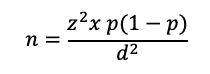Where:
n is the calculated sample size

z is the statistic that defines the level of confidence required

p is an estimate of the key indicator to be measured by the survey in the population group of interest, for example, the prevalence of iron deficiency among WRA, expressed as a proportion of that population

d is the desired level of precision, or the margin of relative error to be obtained.

Generally, Generally, z = 1.96, which is the z-statistic for the 95% confidence level. If the expected estimate of the key indicator (p) is not known, the value of 0.5 (or 50%) is used because it produces the largest sample size (for a given value of d). If the proportion is expected to be between two values, select the value closest to 0.5. For example, if the proportion is thought to be between 0.65 and 0.75, use 0.65 for the sample size calculation.

Common values for d for national level estimates are usually around ±5% for indicators with estimated prevalence in the range of 20%–80% (for example, anaemia), and around ±3% for less common or very common events (for example, wasting, or household coverage of iodized salt). It is often acceptable to use a value of d higher than ±5% at a stratum level, with the knowledge that the precision around the national estimate will be narrower.

## Step 2. Accounting for the Design Effect in Calculating Sample Sizes for Cluster Surveys

Cluster sampling is more feasible than SRS for large-scale surveys because it reduces the number of locations to visit and to map, and in which to set up field laboratories. However, cluster sampling introduces the “clustering effect”, which describes the fact that households in the same cluster tend to be more alike in terms of certain characteristics (for example, income, education and access to a fortified food product) than households across the general population. This clustering effect increases the variance between clusters so that it lowers the precision around an estimate that would have been found based on the same sample size calculated for SRS sampling. This change in precision due to clustering is described as the design effect (DEFF). The DEFF is a measure of the homogeneity within a cluster and the variability between clusters. It indicates how much larger the sampling variance (square of the standard error) is for the cluster sample compared to a simple random sample of the same size.

Unless the DEFF was calculated from previous survey data, it will need to be estimated at the stage of sample size calculation, based on prior field experience (unpublished data or subject matter expertise) or from published literature. Some indicators such as wasting (low weight-for-height) may have a small DEFF, while other indicators such as vitamin A capsule distribution may have a large DEFF. In rare cases, the DEFF may be less than 1, in which case a value of 1 should be used for sample size calculations. The DEFF is calculated using the intra-class correlation (ICC), which is a metric of the similarity of clusters in the outcome of interest. Box 5.4 provides more details on the ICC and how this affects the DEFF.

Calculation of sample size for a cluster survey incorporating the DEFF requires a modified version of the previous equation: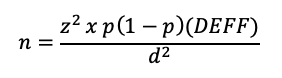There are four important principles that can be applied to keep the DEFF (and sample size) as low as possible:

1. Use as many clusters as is feasible. For a non-stratified survey, the recommended minimum number is 30 clusters in the first stage of sampling. For a stratified survey, the recommended minimum is 25 per stratum. Up to a certain limit, the DEFF will continue to decrease as the number of clusters increases. Sampling more than 40 clusters per stratum does not provide much additional benefit to survey precision, in general.
2. Use the smallest cluster size (number of households per cluster) as is feasible, generally aiming for a minimum of 10 observations for each population group per cluster.
3. Use a constant cluster size.
4. Select a random sample of households at the final stage to increase geographic dispersion (see Module 7: Selecting households and participants).

Note: The DEFF (and hence the precision) for an indicator is affected by the number of observations per cluster, not the number of households visited. As an example, for a sample of 1200 households, a lower DEFF and higher precision would be achieved by selecting 40 clusters of 30 households each instead of 20 clusters of 60 households each.

### Box 5.4. Relationship Between the Design Effect and the Intra-Cluster Correlation

The ICC is a measure of the degree of homogeneity (similarity with respect to the variable of interest) of the units (households or individuals) within a cluster. It is sometimes referred to as the “rate of homogeneity”, or ROH. Because units in the same cluster tend to be similar to one another in terms of income, climate/environmental conditions and attitudes, the ICC is almost always positive. The DEFF can be described in terms of the ICC and vice versa.

For a given deff and average number of units sampled per cluster (n̅), the ICCcan be estimated as: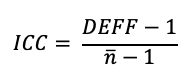If estimates of the ICC and average number of units per cluster are available, the DEFF can be calculated as: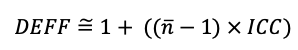The DEFF is significantly affected by the number of observations within one cluster. However, the relationship between the DEFF and the ICC means that the DEFF can be estimated for a subsequent survey that may have a different number of observations per cluster, using the equations above.

For example, analysis of data from the 2015-16 Malawi Micronutrient Status Survey found a DEFF of 1.9 for anaemia among children 6–59 months of age 1. This estimate was based on 105 clusters with an average of 13.8 children per cluster (n=1452). If another survey was being planned with a design to select an average of 16 children per cluster, the ICC-DEFF relationship could be used to estimate the expected DEFF for this second survey.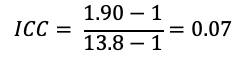The DEFF for the second survey with an average of 16 children per cluster would be estimated as: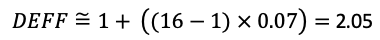Therefore, the estimated DEFF for anaemia for a survey with 16 children per cluster is 2.05, and this would be used in the sample size calculation.

1 National Statistical Office, Community Health Sciences Unit [Malawi], US Centers for Disease Control and Prevention (CDC), Emory University. Malawi Micronutrient Survey 2015-16. Atlanta: US Centers for Disease Control and Prevention; 2017 (https://dhsprogram.com/pubs/pdf/FR319/FR319.m.final.pdf, accessed 20 June 2020).

## Step 3: Accounting for Response Rates

Determining the sample size for household- and individual-level indicators must take into consideration the expected response rates of households and of individuals, and the availability of fortifiable foods. Box 5.2 describes factors to consider. Household and individual response rates can be estimated based on experience from previous similar surveys. Typically, response rates are higher for questionnaire-related indicators, such as an assessment of knowledge or behaviour, and they are lower for food sample collection (usually considered a household-level indicator) and for biological specimens, especially from young children or pregnant women. Ensuring community sensitization to the survey can greatly improve response rates, but when calculating the sample size, it is better to use a relatively conservative estimate.

Non-response can occur at different levels, for example the household interview, individual interview, and food sample and biological specimen collection. Here are potential reasons for non-response:

• None of the household members may be available during the time of the survey (the household is away on a temporary basis).
• The entire household may choose not to participate.
• Some individuals within a household may refuse to participate, may be sick, or may not be available during the survey.
• Some individuals may partially participate, such as agreeing to answer questions but refusing to provide a food sample or biological specimen collection.
• The fortifiable food of interest may not be available for collection within the household.
• The volume of blood or food sample collected may be insufficient for laboratory analysis.
All of these possible reasons for non-participation or non-response should be taken into consideration. The final number of households to be visited to obtain data for the required number of participants from a specific population group may be calculated as: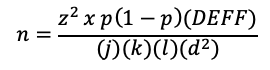Where:
j is the expected response rate as a proportion (household response multiplied by individual response for interview/biological specimen collection)

k is the average household size

l is the proportion of the total population accounted for by the population group of interest

d is the desired level of precision, or the margin of relative error to be obtained

DEFF is the design effect

z is the statistical value derived from the normal distribution table for a given level of confidence (for example, z=2.58 when the type I error, or level of significance: alpha (α)=0.01).

An example calculation for one indicator is shown in Box 5.5.

The ”Survey sample size calculator” online tool can facilitate the process of sample size calculation and makes it easy to see, in the above equation, the effect of changing precision or other variables on final sample size. Additional computer-based programs to perform sample size calculations can be found at www.OpenEpi.com.

There may be differences between hand-calculated sample size estimates and computer-based calculations due to rounding and slight variations in sample size formulae, but these differences are usually minor.

### Box 5.5. Example Sample Size Calculation

The required (unit) sample size to assess vitamin A deficiency among children 6–59 months of age was calculated assuming p = 0.35 (prevalence of deficiency 35%), level of acceptable precision d =.05 (or ±5%), and DEFF = 2.5: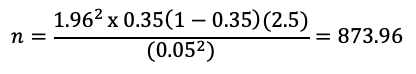Sample sizes are always rounded up, so this would be a desired sample size of 874 children.

The average household size is 3.9 and the proportion of this age group in the population is 0.09, while the household response rate is 90% and the individual response rate is 85%. In order to achieve the target sample size of 874 children, 3255 households should be visited.Decreasing the acceptable level of precision by 2% reduces the number of households to visit by more than 1500. Using an acceptable precision of 0.07, or ±7%, results in a household sample size of 1661 households.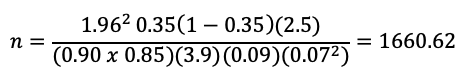Depending on the setting, different precision values or different expected DEFF values may be appropriate. For example, an estimated household coverage of adequately iodized salt of 20% would indicate significant problems with implementing universal salt iodization. It could be decided that a precision of ±10% would be sufficient because the programme response would be the same whether the true prevalence was anywhere between 10% and 30%. On the other hand, if the prevalence of the indicator of interest is very low or very high, or expected to be close to the cutoff value for public health significance, it may be desirable to have a precision of ±5% or, in rare cases, an even higher precision of ±2.5%. However, the impact of such increased precision on sample size must be weighed against the programmatic application of the outcome.

1. Urinary iodine concentrations for determining iodine status in populations. Vitamin and Mineral Nutrition Information System. Geneva: World Health Organization; 2013 (WHO/NMH/NHD/EPG/13.1; https://apps.who.int/iris/bitstream/handle/10665/85972/WHO_NMH_NHD_EPG_13.1_eng.pdf, accessed 15 June 2020).

## Calculating anthropometry Z-scores igrowup SAS

SAS code to calculate Z-scores

## Survey Sample Size Calculator

Sample size calculation spreadsheet for single and multiple cross sectional surveys